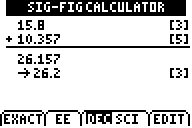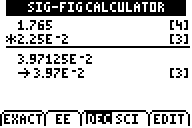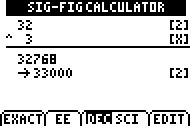# Knowledge Base

## Solution 30190: Using The Significant Numbers Calculator From The Science Tools App on the TI-83 Plus and TI-84 Plus Family of Graphing Calculators.

### How do I use the Significant Numbers calculator from the Science Tools App?

Calculations that involve measurements include a certain amount of error. The precision of the measurement determines which digits (known as significant digits or significant figures) should be included when you report measurement results. However, you will often encounter measurement values reported with arbitrary precision. The Sig-Fig Calculator tool lets you:

• Display the significant figures of entered values.
• Perform mathematical operations using either decimal or scientific notation and display the result with the correct number of significant digits.
• Convert entries in decimal notation to scientific notation.
• Convert entries in scientific notation to decimal notation.

Open the Science Tools application by pressing the [APPS] key, using the arrow keys to highlight SciTools, then pressing [ENTER]. From the Science Tools menu, choose the Sig-Fig Calculator. The number of significant figures displayed in brackets to the right of the processed result matches the number of significant figures actually displayed in the final processed value. The exponent function of the Sig-Fig Calculator tool is intended for integer exponents only. Entering non-integer exponents may produce unexpected results.

Enter the numbers and operators in the order the operations should be performed.
Press [ENTER] to calculate the result. The result is rounded to the correct number of significant figures.

For addition and subtraction, the rounded result has the same decimal-place precision as the least precise term.For multiplication and division, the rounded result has the same number of significant figures as the factor with the least number of significant figures.For powers, the rounded result has the same number of significant figures as the base.You can use any combination of addition, subtraction, multiplication, division and raising a value to a power. Multiplication and division are performed first, and then addition and subtraction are performed.

You cannot use parentheses in the Sig-Fig Calculator. To perform calculations that would normally include parentheses, you must perform the operations separately.

Some values, such as defined or counted quantities, are exact and should not be rounded and should not affect the rounding of other numbers. To set a value as exact:

1) Enter the value in the Sig-Fig Calculator.

2) Select "Exact" (which corresponds to the [Y=] key).

To enter a value in scientific notation:

1) Enter the number.

2) Select "EE" (which corresponds to the [WINDOW] key).

DEC (decimal) or SCI (scientific) is highlighted to indicate the current mode.  Press [ZOOM] or [TRACE] to change the mode. The mode change does not apply to a calculation currently displayed; it applies to the next entry you make. You can correct mistakes using the "Edit" option, even after the calculation has been performed.

3) Select "Edit" (which corresponds with the [GRAPH] key).

The result is removed and the cursor moves to the end of the top line. For more information, please see the Science Tools Guidebook.